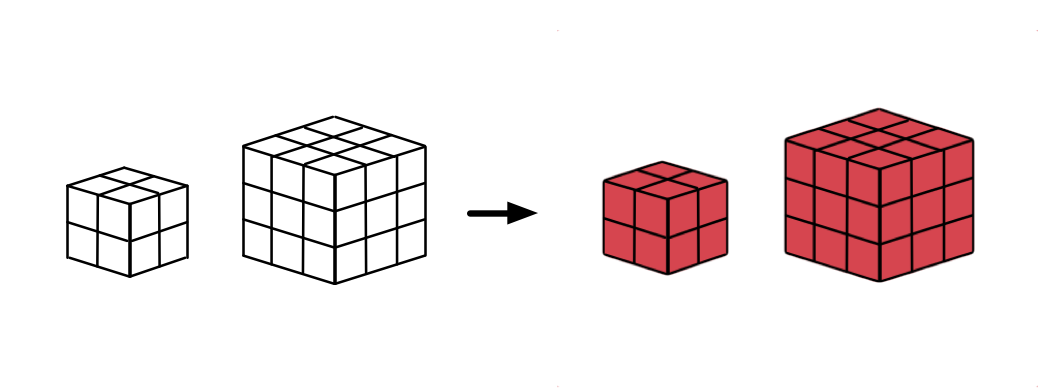# Unit Cubes 5 – $n \times n$

Probability Level 1On an $n \times n \times n$ cube made out of $1 \times 1 \times 1$ unit cubes (with $n \geq 2$ ) with the exterior painted red, how many of the unit cubes will have at least one red face?

×